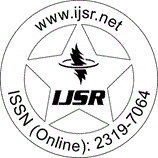International Journal of Science and Research (IJSR)
Call for Papers | Fully Refereed | Open Access | Double Blind Peer Reviewed

Research Paper | Mathematics | India | Volume 4 Issue 3, March 2015

# Finite Difference Approximation for Transport Equation with Shifts Arising in Neuronal Variability

Chhatra Pal  | Agam Dwivedi

Abstract: This paper studies some finite difference approximations to find the numerical solution of first-order hyperbolic partial differential equation of mixed type, i. e. , transport equation with point-wise delay and advance. We are interested in the challenging issues in neuronal science stemming from the modeling of neuronal variability based on Steins Model . The resulting mathematical model is a first order hyperbolic partial differential equation having point-wise delay and advance which models the distribution of time intervals between successive neuronal firings. We construct, analyze and implement explicit numerical scheme for solving such type of initial and boundary-interval problems. Analysis shows that numerical scheme is conditionally stable, consistent and convergent in discrete L infinity norm. Some numerical tests are reported to validate the computational efficiency of the numerical approximation.

Keywords: hyperbolic partial differential equation, neuronal firing, point-wise delay and advance, finite difference method, Lax- Friedrichs scheme

Edition: Volume 4 Issue 3, March 2015,

Pages: 2238 - 2242